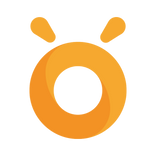Back to scenarios
3, 4, 5Author:

# Number and Operations in Base Ten, Photon Knows The Place

Mathematik
Duration time:
60 min.
Robots:
x3+
Programming interface:
Photon DrawPhoton BlocksScratch 3.0Back to scenariosShare
Share

## Reference to the core curriculum

```Common Core Math Standards:
- CCSS.MATH.CONTENT.3.NBT.A.1: Use place value understanding to round whole numbers to the nearest 10 or 100.
- CCSS.MATH.CONTENT.4.NBT.A.2: Read and write multi-digit whole numbers using base-ten numerals, number names, and expanded form. Compare two multi-digit numbers based on meanings of the digits in each place, using >, =, and < symbols to record the results of comparisons.
- CCSS.MATH.CONTENT.4.NBT.A.3: Use place value understanding to round multi-digit whole numbers to any place.
- CCSS.MATH.CONTENT.5.NBT.A.3.B: Compare two decimals to thousandths based on meanings of the digits in each place, using >, =, and < symbols to record the results of comparisons.
- CCSS.MATH.CONTENT.5.NBT.A.4: Use place value understanding to round decimals to any place.```

## Class goal:

Objectives:
• To round whole numbers or decimals to the selected place value.
• To compare two multi-digit numbers or decimals to thousandths.

• Group work.

## Required items:

• One Photon Robot and devices for programming per every two to three students.
• Individual digits written on index cards (each group will need a set).

## Lesson scenario:

Programming Interface: Photon Draw, Photon Blocks or Scratch via the Photon EDU App or the Photon Magic Bridge.

Preparation:

• The teacher will prepare the individual digits on index cards. There should be enough of each digit to lay them on the floor to create the numbers the teacher has selected to use in the lesson. (one set per group)
• The teacher will have the selected numbers to round or compare prepared to display during the activity.
• The teacher will instruct the students on rounding numbers and/or comparing numbers.
• Break the students into groups of two to three students.
• Explain how students will code Photon to show their answer to rounding a number.
• Instruct the students about which place values they will be rounding to.
• Explain that Photon should be programmed to drive to the place value that is being rounded to and turn his ears red.  Then, he should be programmed to drive to the digit next to it.  Using the record feature, the students should record “the digit _____ means stay or go up”.  Example:  “Three means stay.”  Photon should be driven back to the place value being rounded to and the students should program him to read the rounded number.
• Explain how students will code Photon to give their answer when comparing numbers.
• Explain that students should program Photon to show whether the first number is greater than, less than, or equal to the second number.
• They will use a code that the class creates together.

Lesson Sequence:

Rounding

• Each group will be working together to round the number.
• Review how to code Photon to show their work.
• Have each group get a Photon and coding device and go to a space where they can place their digits on the floor and discuss their answer as they program Photon.
• Display the first number each group should round.
• In their groups, students work to program Photon to show their answer.
• After all the groups have coded their robot to show their thinking and read the rounded number, each group should be asked to run their program.
• Answer any questions or clear up any misunderstandings.
• Repeat for each number.

Comparing

• Each group will be working together to compare the numbers.
• Work with the students to determine how they will show <, >, or =. Example:  Select a different sound for each symbol, select a different color for each symbol, or determine a movement sequence for each symbol.
• Display the code selected to represent each symbol.
• Have each group get a Photon and coding device and go to a space where they can place their digits on the floor and discuss their answer as they program Photon.
• Display the first two numbers that should be compared.
• In their groups, students work to program Photon to show their answer.
• After all the groups have coded their robot to show their answer, each group should be asked to run their program.